Processing ......FreeComputerBooks.com Links to Free Computer, Mathematics, Technical Books all over the World

Number Theory: In Context and Interactive
🌠 Top Free Unix/Linux Books - 100% Free or Open Source!
• Title: Number Theory: In Context and Interactive
• Author(s) Karl-Dieter Crisman
• Publisher: Independently published (Jan. 15 2021); eBook (Creative Commons Licensed, July, 2021)
• Hardcover/Paperback: 477 pages
• eBook: HTML and PDF (510 pages)
• Language(s): English
• ISBN-10/ASIN: B08XLGFMNR
• ISBN-13: 979-8713540685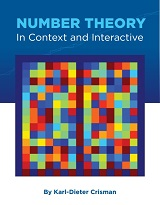Book Description

This is a textbook for an upper-level (US) number theory course, with a clear vision to expose students to the connections to all areas of mathematics.

There are many exercises, both proof-based and computational, and nearly every concept can be visualized or experimented with using the open source mathematics software SageMath.

The book tackles all standard topics of modular arithmetic, congruences, and prime numbers, including quadratic reciprocity. In addition, there is significant coverage of various cryptographic issues, geometric connections, arithmetic functions, and basic analytic number theory, ending with a beginner's introduction to the Riemann Hypothesis.

Ordinarily this should be enough material for a semester course with no prerequisites other than a proof-transition experience and vaguely remembering some calculus.

• Karl-Dieter Crisman is a Professor of Mathematics at Gordon College, Massachusetts, United States.
Reviews, Ratings, and Recommendations: Related Book Categories: Read and Download Links:Similar Books:
•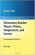Elementary Number Theory: Primes, Congruences, and Secrets

This is a book about prime numbers, congruences, secret messages, and elliptic curves that you can read cover to cover. Many numerical examples are given throughout the book using the SageMath mathematical software.

•An Introduction to the Theory of Numbers (Leo Moser)

This book, which presupposes familiarity only with the most elementary concepts of arithmetic (divisibility properties, greatest common divisor, etc.), is an expanded version of a series of lectures for graduate students on elementary number theory.

•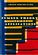Number Theory and Its Applications (Cheon Seoung Ryoo)

Number theory plays a fundamental and important role in mathematics and applied mathematics. This book is based on recent results in all areas related to number theory and its applications.

•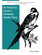An Introductory Course in Elementary Number Theory (Wissam Raji)

This book contains a useful introduction to important topics that need to be addressed in a course in number theory. Proofs of basic theorems are presented in an interesting and comprehensive way that can be read and understood even by non-majors.

•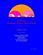Yet Another Introductory Number Theory Textbook (J. A. Poritz)

This introductory number theory textbook has a particular emphasis on connections to cryptology. It broaches the subject of these cryptologic algorithms to develop connections to the topic of number theory.

•Topology of Numbers (Allen Hatcher)

A textbook on elementary number theory from a geometric point of view, as opposed to the usual strictly algebraic approach. A fair amount of the book is devoted to studying Conway's topographs associated to quadratic forms in two variables.

•Magic Squares and Cubes (William Symes Andrews)

This book cover topics such as magic squares, magic cubes, the Franklin squares, magics and Pythagorean numbers, the theory of reversions, magic circles, spheres, and stars, and magic octahedroids, among other things.

•A Computational Introduction to Number Theory and Algebra

This introductory book emphasises algorithms and applications, such as cryptography and error correcting codes, and is accessible to a broad audience. The coverage includes the basics of number theory, abstract algebra and discrete probability theory.

•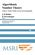Algorithmic Number Theory: Lattices, Curves, and Cryptography

This text provides a comprehensive introduction to algorithmic number theory for beginning graduate students, written by the leading experts in the field. It includes several articles that cover the essential topics in this area.

•A Course in Algebraic Number Theory (Robert B. Ash)

This book explores the general theory of factorization of ideals in Dedekind domains as well as the number field case. Detailed calculations illustrate the use of Kummer's theorem on lifting of prime ideals in extension fields.

•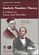Analytic Number Theory: A Tribute to Gauss and Dirichlet

The book begins with a definitive summary of the life and work of Dirichlet and continues with thirteen papers by leading experts on research topics of current interest in number theory that were directly influenced by Gauss and Dirichlet.

Book Categories
 :All CategoriesTop Free BooksRecent BooksMiscellaneous BooksComputer EngineeringComputer LanguagesComputer ScienceData Science/DatabasesJava and Java EE (J2EE)Linux and UnixMathematicsMicrosoft and .NETMobile ComputingNetworking and CommunicationsSoftware EngineeringSpecial TopicsWeb Programming
Other Categories